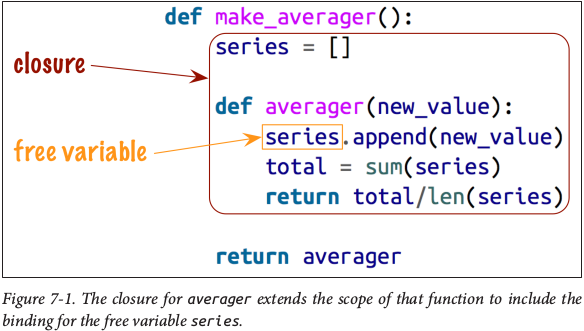# TAPP1: Decorator and Closure

### 为什么需要装饰器(MotivaMotion)¶

#### 计时器,日志记录¶

def func():
print("Hello, I am func.")


import time

def func():
start = time.time()
print("Hello, I am func.")
end = time.time()
print('Spend:{}s'.format(end - start))

if __name__ == '__main__':
func()


Hello, I am func.
Spend:2.5510787963867188e-05s


import time

def func():
print("Hello, I am a function.")

if __name__ == '__main__':
start = time.time()
func()
end = time.time()
print('Spend:{}s'.format(end - start))


Hello, I am a function.
Spend:2.2411346435546875e-05s


import time

def timeIt(func):
def wrapper():
start = time.time()
func()
end = time.time()
print('Spend:{}s'.format(end - start))
return wrapper

@timeIt
def func1():
print("Hello, I am func1.")

@timeIt
def func2():
print("Hello, I am func2.")

if __name__ == '__main__':
func1()
func2()


Hello, I am func1.
Spend:3.886222839355469e-05s
Hello, I am func2.
Spend:8.106231689453125e-06s


### 装饰器是什么¶

"A decorator is a callable that takes a callable as input and returns another callable." -- Dan, Python Tricks: The Book

NOTE

### 什么是闭包¶

“Actually, a closure is function with an extended scope that encompasses non-global variables referenced in the body of the function but not defined there.” ——Fluent Python

def make_averager():
series = []

def averager(new_value):
series.append(new_value)
total = sum(series)

return averager>>> avg.__code__.co_freevars
('series',)
>>> avg.__closure__
(<cell at 0x7ff64747f978: list object at 0x7ff6475695c8>,)
>>> avg.__closure__.cell_contents
[10, 11]


### 修改闭包中自由变量¶

def make_averager():
count = 0
total = 0

def averager(new_value):
count += 1
total += new_value
reutrn total / count
return averager

>>> avg = make_averager()
>>> avg(10)
Traceback (most recent call last):
File "<stdin>", line 1, in <module>
File "test.py", line 6, in averager
count += 1
UnboundLocalError: local variable 'count' referenced before assignment


def make_averager():
count = 0
total = 0

def averager(new_value):
nonlocal count, total
count += 1
total += new_value
return averager


### 怎么用装饰器¶

#### 如何写&作用原理¶

def timeIt(func):
def wrapper():
start = time.time()
result = func()
end = time.time()
print('Spend:{}s'.format(end - start))
return result
return wrapper


@timeIt
def func1():
print("Hello, I am func1.")

func1 = timeIt(func1)


#### 参数传入(被装饰函数func)¶

import time

def timeIt(func):
def wrapper(*args, **kwargs):
start = time.time()
result = func(*args, **kwargs)
end = time.time()
print('Spend:{}s'.format(end - start))
return result
return wrapper

@timeIt
def func_args(x, y, a=3):
print("Hello, I have three args: %s, %s, %s." % (x, y, a))

>>> func_args(1, 2)
Hello, I have three args: 1, 2, 3.
Spend:5.602836608886719e-05s


#### 参数传入(装饰器本身timeIt)¶

import time

def timeIt(func, seconds=True):
# 以秒为单位，返回浮点数
if seconds:
measure = time.perf_counter
fmt = 'Spend:{}s'
else:
# 以纳秒为单位，返回整数
measure = time.perf_counter_ns
fmt = 'Spend:{}ns'

def wrapper(*args, **kwargs):
start = measure()
result = func(*args, **kwargs)
end = measure()
print(fmt.format(float(end) - float(start)))
return result
return wrapper

@timeIt(seconds=False)
def func_args(x, y, a=3):
print("Hello, I have three args: %s, %s, %s." % (x, y, a))


Traceback (most recent call last):
File "test.py", line 23, in <module>
@timeIt(seconds=False)
TypeError: timeIt() missing 1 required positional argument: 'func'


import time

def timeIt(func, seconds=True):
# 以秒为单位，返回浮点数
if seconds:
measure = time.perf_counter
fmt = 'Spend:{}s'
else:
# 以纳秒为单位，返回整数
measure = time.perf_counter_ns
fmt = 'Spend:{}ns'

def wrapper(*args, **kwargs):
start = measure()
result = func(*args, **kwargs)
end = measure()
print(fmt.format(float(end) - float(start)))
return result
return wrapper

def func_args(x, y, a=3):
print("Hello, I have three args: %s, %s, %s." % (x, y, a))

>>> func1 = timeIt(func_args, seconds=True)
>>> func1(1, 2)
Hello, I have three args: 1, 2, 3.
Spend:3.587399987736717e-05s
>>> func2 = timeIt(func_args, seconds=False)
>>> func2(1, 2)
Hello, I have three args: 1, 2, 3.
Spend:64493.0ns


import time

def clock(seconds=True):
# 以秒为单位，返回浮点数
if seconds:
measure = time.perf_counter
fmt = 'Spend:{}s'
else:
# 以纳秒为单位，返回整数
measure = time.perf_counter_ns
fmt = 'Spend:{}ns'

def timeIt(func):
def wrapper(*args, **kwargs):
start = measure()
result = func(*args, **kwargs)
end = measure()
print(fmt.format(float(end) - float(start)))
return result
return wrapper
return timeIt

@clock()
def func_args_1(x, y, a=3):
print("Hello, I have three args: %s, %s, %s." % (x, y, a))

@clock(seconds=False)
def func_args_2(x, y, a=3):
print("Hello, I have three args: %s, %s, %s." % (x, y, a))

>>> func_args_1(1, 2)
Hello, I have three args: 1, 2, 3.
Spend:8.46920011099428e-05s
>>> func_args_2(1, 2)
Hello, I have three args: 1, 2, 3.
Spend:62385.0ns


#### 多重装饰(decorator stacking)¶

def add_hi(func):
def wrapper(*args, **kwargs):
org = func(*args, **kwargs)
return '<hi>' + org + '</hi>'
return wrapper

def wrapper(*args, **kwargs):
org = func(*args, **kwargs)
return '<hello>' + org + '</hello>'
return wrapper

def func():
return 'func'

>>> func()
'<hello><hi>func</hi></hello>'


@add_hello
def func():
return 'func'

func = add_hello(add_hi(func))


#### 可用于DEBUG的装饰器¶

def add_hello(func):
def wrapper(*args, **kwargs):
org = func(*args, **kwargs)
return '<hello>' + org + '</hello>'
return wrapper

def func():
"""
Just return the name of the function.
"""
return 'func'


>>> func.__name__
'func'
>>> func.__doc__
'Just return the name of the function.'
>>> decorate_func.__name__
'wrapper'
>>> decorate_func.__doc__
>>>


import functools

@functools.wraps(func)
def wrapper(*args, **kwargs):
org = func(*args, **kwargs)
return '<hello>' + org + '</hello>'
return wrapper

def func():
"""Just return the name of the function."""
return 'func'

>>> func.__name__
'func'
>>> func.__doc__
'Just return the name of the function.'


#### 注意事项¶

decorator stacking虽好，也没有层次限制，但是当堆叠太多的时候还是对程序的运行效率带来压力，毕竟本身是函数的调用。

NOTE Python也是有Class Decorator的哦，后面可能会讲到:-)

A common use of class decorators is to be a simpler alternative to some use-cases of metaclasses. In both cases, you are changing the definition of a class dynamically. (Primer on Python Decorators)